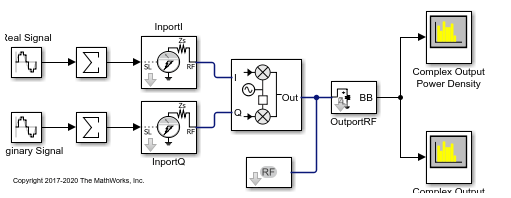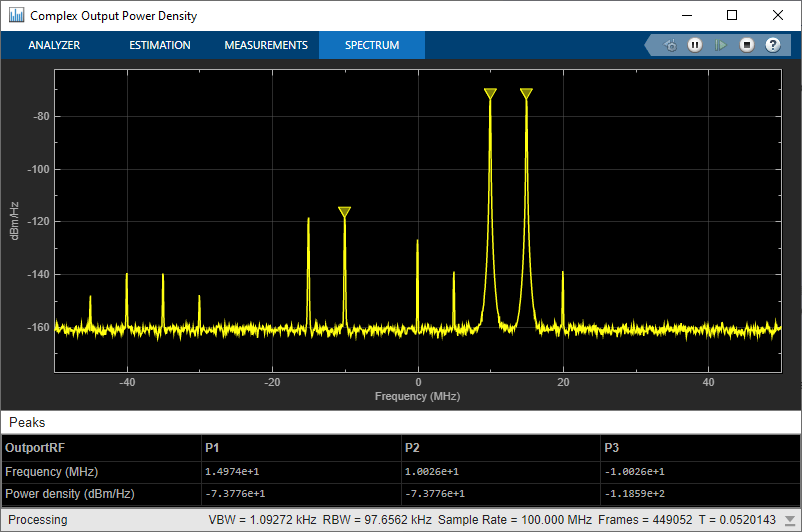# Modulate Two-Tone DC Signal Using IQ Modulator

Use the IQ Modulator block to Modulate a two-tone signal to RF level. Observe the impairments in the modulated output signal such as images due to gain imbalance, intermodulation distortion, and output third-order intercept (OIP3).

The two-tones are at 10 MHz and 15 MHz.The power of each tone is -30 dBm. The carrier frequency is 0 GHz.

### IQ Modulator

The IQ modulator parameters are :

• Available power gain: 10 dB

• Local oscillator frequency: 2 GHz

• I/Q gain mismatch: 0.1 dB

• LO to RF Isolation: 90 dB

• Noise Floor: -160 dBm/Hz

• IP3: 10 dBm

Open the model.

```open('model_IQmod') ```Run the model and observe the spectrum analyzers.

### Complex Output Power DensityIn the complex output power density spectrum analyzer, you see the noise floor of the signal at `-160` dBm/Hz.

### Complex Output Spectrum AnalyzerIn the complex output spectrum analyzer, you see the whole modulated signal including the imaginary parts. The output power level of the two tones (10 MHz and 15 MHz)is -20 dBm.

``

The output third-order intercept( OIP3) is at 10 dBm. The spectrum analyzer measures this value.

### Image Rejection Ratio

The images of the two-tones are at `-10` MHz and `-15` MHz. The output power level of the two images are `-67.8` dBm. Image rejection ratio is calculated using this formula:

``

where,

``
``

The image power level is calculated using this:

``